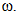# In a uniform magnetic field of induction B a wire in the form of a semicircle of radius r rotates about the diameter of the circle with angular f...

In a uniform magnetic field of induction B a wire in the form of a semicircle of radius r rotates about the diameter of the circle with angular frequency. The axis of rotation is perpendicular to the field. If the total resistance of the circuit is R the mean power generated per period of rotation is

Anonymous User Physics Magnetostatics 19 Apr, 2020 57 views